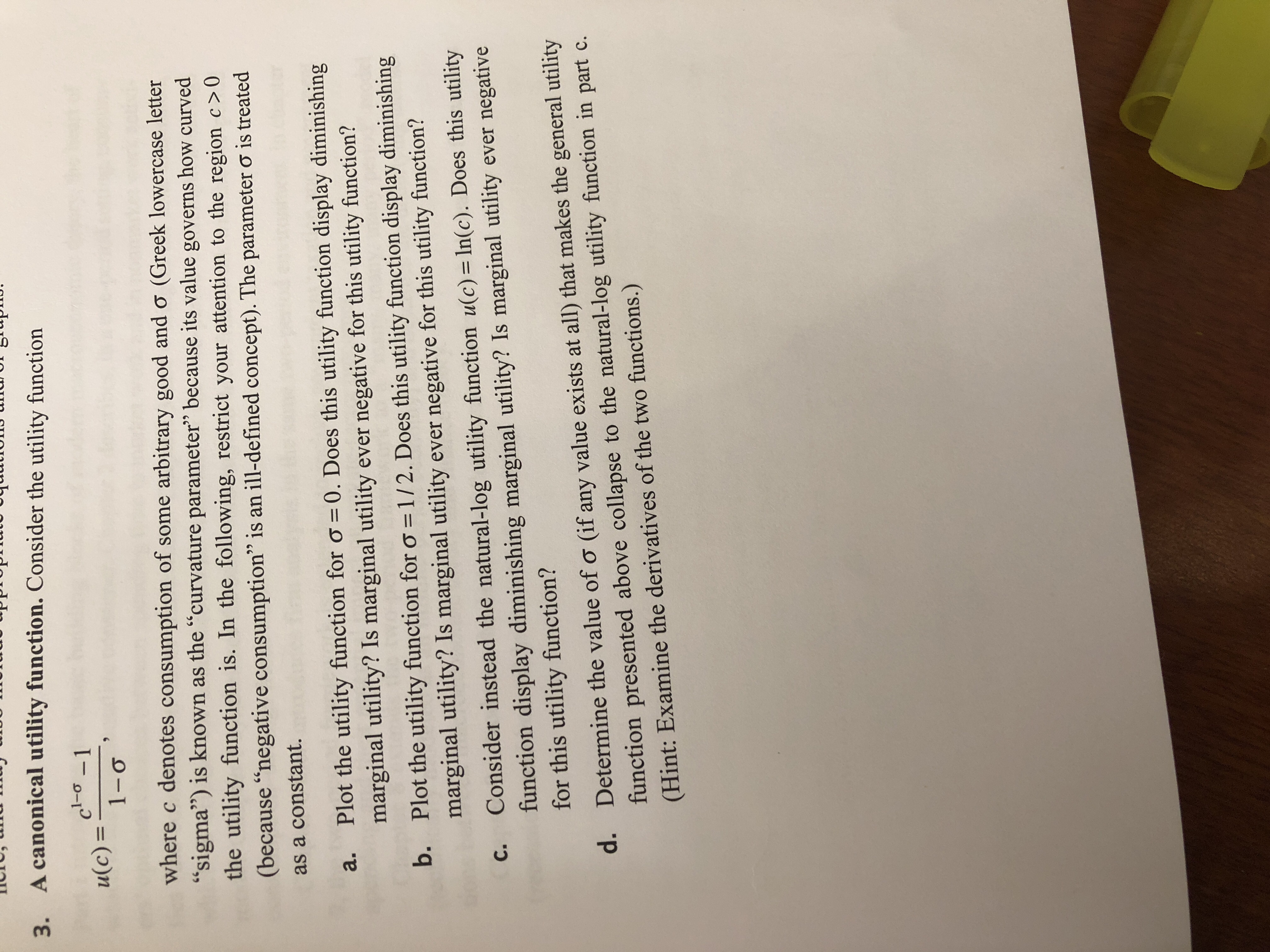# 3. A canonical utility function. Consider the utility function u(c) 1-σ where c denotes consumption of some arbitrary good and ơ (Greek lowercase letter sigma") is known as the "curvature parameter" because its value governs how curved the utility function is. In the following, restrict your attention to the region c> (because "negative consumption" is an ill-defined concept). The parameter σ is treated as a constant. Plot the utility function for ơ-0. Does this utility function display diminishing marginal utility? Is marginal utility ever negative for this utility function? Plot the utility function for ơ marginal utility? Is marginal utility ever negative for this utility function? Consider instead the natural-log utility function u(c)=In(c). Does this utility function display diminishing marginal utility? Is marginal utility for this utility function? Determine the value of σ (if any value exists at all) that makes the general utility function presented above collapse to the natural-log utility function in part c. (Hint: Examine the derivatives of the two functions.) a. b. 2. Does this utility function display diminishing c. ever negative d.

Question
105 viewshelp_outlineImage Transcriptionclose3. A canonical utility function. Consider the utility function u(c) 1-σ where c denotes consumption of some arbitrary good and ơ (Greek lowercase letter sigma") is known as the "curvature parameter" because its value governs how curved the utility function is. In the following, restrict your attention to the region c> (because "negative consumption" is an ill-defined concept). The parameter σ is treated as a constant. Plot the utility function for ơ-0. Does this utility function display diminishing marginal utility? Is marginal utility ever negative for this utility function? Plot the utility function for ơ marginal utility? Is marginal utility ever negative for this utility function? Consider instead the natural-log utility function u(c)=In(c). Does this utility function display diminishing marginal utility? Is marginal utility for this utility function? Determine the value of σ (if any value exists at all) that makes the general utility function presented above collapse to the natural-log utility function in part c. (Hint: Examine the derivatives of the two functions.) a. b. 2. Does this utility function display diminishing c. ever negative d. fullscreen

### Want to see the step-by-step answer?